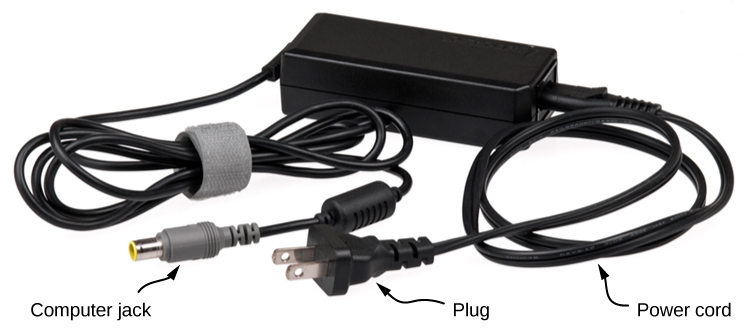# 5.2 Conductors, insulators, and charging by induction

 Page 1 / 10
By the end of this section, you will be able to:
• Explain what a conductor is
• Explain what an insulator is
• List the differences and similarities between conductors and insulators
• Describe the process of charging by induction

In the preceding section, we said that scientists were able to create electric charge only on nonmetallic materials and never on metals. To understand why this is the case, you have to understand more about the nature and structure of atoms. In this section, we discuss how and why electric charges do—or do not—move through materials ( [link] ). A more complete description is given in a later chapter.This power adapter uses metal wires and connectors to conduct electricity from the wall socket to a laptop computer. The conducting wires allow electrons to move freely through the cables, which are shielded by rubber and plastic. These materials act as insulators that don’t allow electric charge to escape outward. (credit: modification of work by “Evan-Amos”/Wikimedia Commons)

## Conductors and insulators

As discussed in the previous section, electrons surround the tiny nucleus in the form of a (comparatively) vast cloud of negative charge. However, this cloud does have a definite structure to it. Let’s consider an atom of the most commonly used conductor, copper.

For reasons that will become clear in Atomic Structure , there is an outermost electron that is only loosely bound to the atom’s nucleus. It can be easily dislodged; it then moves to a neighboring atom. In a large mass of copper atoms (such as a copper wire or a sheet of copper), these vast numbers of outermost electrons (one per atom) wander from atom to atom, and are the electrons that do the moving when electricity flows. These wandering, or “free,” electrons are called conduction electron     s , and copper is therefore an excellent conductor    (of electric charge). All conducting elements have a similar arrangement of their electrons, with one or two conduction electrons. This includes most metals.

Insulators , in contrast, are made from materials that lack conduction electrons; charge flows only with great difficulty, if at all. Even if excess charge is added to an insulating material, it cannot move, remaining indefinitely in place. This is why insulating materials exhibit the electrical attraction and repulsion forces described earlier, whereas conductors do not; any excess charge placed on a conductor would instantly flow away (due to mutual repulsion from existing charges), leaving no excess charge around to create forces. Charge cannot flow along or through an insulator    , so its electric forces remain for long periods of time. (Charge will dissipate from an insulator, given enough time.) As it happens, amber, fur, and most semi-precious gems are insulators, as are materials like wood, glass, and plastic.

## Charging by induction

Let’s examine in more detail what happens in a conductor when an electrically charged object is brought close to it. As mentioned, the conduction electrons in the conductor are able to move with nearly complete freedom. As a result, when a charged insulator (such as a positively charged glass rod) is brought close to the conductor, the (total) charge on the insulator exerts an electric force on the conduction electrons. Since the rod is positively charged, the conduction electrons (which themselves are negatively charged) are attracted, flowing toward the insulator to the near side of the conductor ( [link] ).

calculate ideal gas pressure of 0.300mol,v=2L T=40°c
what is principle of superposition
what are questions that are likely to come out during exam
what is electricity
watt is electricity.
electricity ka full definition with formula
Jyoti
If a point charge is released from rest in a uniform electric field will it follow a field line? Will it do so if the electric field is not uniform?
Maxwell's stress tensor is
Yes
doris
neither vector nor scalar
Anil
if 6.0×10^13 electrons are placed on a metal sphere of charge 9.0micro Coulombs, what is the net charge on the sphere
18.51micro Coulombs
ASHOK
Is it possible to find the magnetic field of a circular loop at the centre by using ampere's law?
Is it possible to find the magnetic field of a circular loop at it's centre?
yes
Brother
The density of a gas of relative molecular mass 28 at a certain temperature is 0.90 K kgmcube.The root mean square speed of the gas molecules at that temperature is 602ms.Assuming that the rate of diffusion of a gas in inversely proportional to the square root of its density,calculate the density of
A hot liquid at 80degree Celsius is added to 600g of the same liquid originally at 10 degree Celsius. when the mixture reaches 30 degree Celsius, what will be the total mass of the liquid?
Under which topic
doris
what is electrostatics
Study of charges which are at rest
himanshu
Explain Kinematics
Two equal positive charges are repelling each other. The force on the charge on the left is 3.0 Newtons. Using your notes on Coulomb's law, and the forces acting on each of the charges, what is the force on the charge on the right?
Using the same two positive charges, the left positive charge is increased so that its charge is 4 times LARGER than the charge on the right. Using your notes on Coulomb's law and changes to the charge, once the charge is increased, what is the new force of repulsion between the two positive charges?
Nya
A mass 'm' is attached to a spring oscillates every 5 second. If the mass is increased by a 5 kg, the period increases by 3 second. Find its initial mass 'm'
a hot water tank containing 50,000g of water is heated by an electric immersion heater rated at 3kilowatt,240volt, calculate the currentBy Katherina jennife...By David GeltnerBy Jugnu KhanBy RhodesBy John GabrieliBy OpenStaxBy OpenStaxBy Jonathan LongBy David MartinBy Jonathan Long